Importance: High ✭✭✭
 Subject: Graph Theory
 Keywords: chromatic number, fractional power of graph, clique number
 Posted by: Iradmusa on: April 23rd, 2011
Conjecture   Letbe a graph andbe a positive integer. Thepower of, denoted by, is defined on the vertex set, by connecting any two distinct verticesand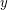with distance at most. In other words,. Alsosubdivision of, denoted by, is constructed by replacing each edge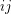ofwith a path of length. Note that for, we have.
Now we can define the fractional power of a graph as follows:
Letbe a graph and. The graph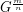is defined by thepower of thesubdivision of. In other words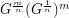.
Conjecture. Letbe a connected graph withandbe a positive integer greater than 1. Then for any positive integer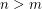, we have.
In , it was shown that this conjecture is true in some special cases.

## Bibliography

 Iradmusa, Moharram N., On colorings of graph fractional powers. Discrete Math. 310 (2010), no. 10-11, 1551–1556.

* indicates original appearance(s) of problem.